# Electrical Mapping

See code in GitLab.
Author: Fernando Campos fernando.campos@kcl.ac.uk

This example demonstrates how to compute local activation times (LATs) and action potential durations (APDs) of cells in a cardiac tissue. To run the experiments of this example change directories as follows:

cd ${TUTORIALS}/02_EP_tissue/08_lats ## Electrical Mapping The wavelength of the cardiac impulse, given by the product of conduction velocity (CV) and refractory period, is of utmost importance for the study of arrhythmia mechanisms. The assessment of the CV as well as the refractory period of the cardiac action potential (AP) relies on the determination of activation and repolarization times, respectively. Experimentally, these are usually calculated based on 1) transmembrane voltages $$V_{\mathrm m}$$ measured by glass micro-electrodes or in optical mapping; or 2) extracellular potentials $$\phi_{\mathrm e}$$ measured at the surface of the tissue. The estimation of activation/repolarization times is based on an event detector that records the instants when the selected event type occurs. Local activation time (LAT), for instance, is usually taken as the time of maximum $$\mathrm{d}V_{\mathrm m}/\mathrm{d}t$$ or the minimum $$\mathrm{d}\phi_{\mathrm e}/\mathrm{d}t$$: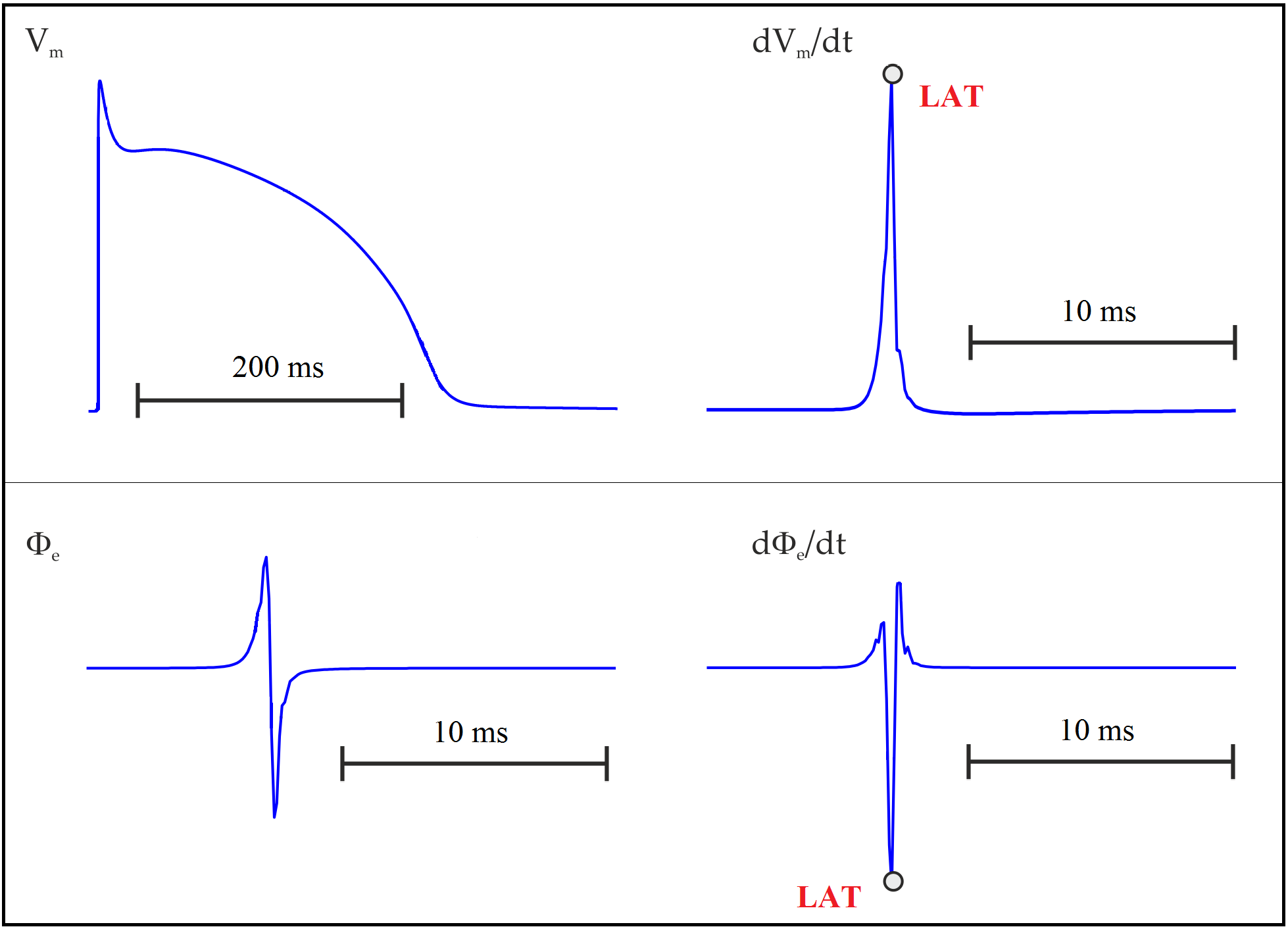Action potential in an uncoupled cell. Upper panel: transmembrane potential $$V_{\mathrm m}$$ and its time derivative $$\mathrm{d}V_{\mathrm m}/\mathrm{d}t$$. Lower panel: extracellular potential $$\phi_{\mathrm e}$$ and its time derivative $$\mathrm{d}\phi_{\mathrm e}/\mathrm{d}t$$. LAT is usually taken as the time of maximum $$\mathrm{d}V_{\mathrm m}/\mathrm{d}t$$ or the minimum $$\mathrm{d}\phi_{\mathrm e}/\mathrm{d}t$$. Instead of using derivatives, LATs can also been estimated by the crossing of a threshold value provided that the chosen value reflects the sodium channel activation since they are responsible for the rising phase of AP. One could, for instance, pick the time instant when $$V_{\mathrm m}$$ crosses -10 mV with a positive slope. Equally, one could look for crossing of $$V_{\mathrm m}$$ with a negative slope to detect repolarization events. AP duration (APD) can then be computed as the difference between repolarization and activation times. The detection of activation ($$t_{\mathrm{act}}$$) and repolarization ($$t_{\mathrm{rep}}$$) times based on threshold crossing is illustrated below: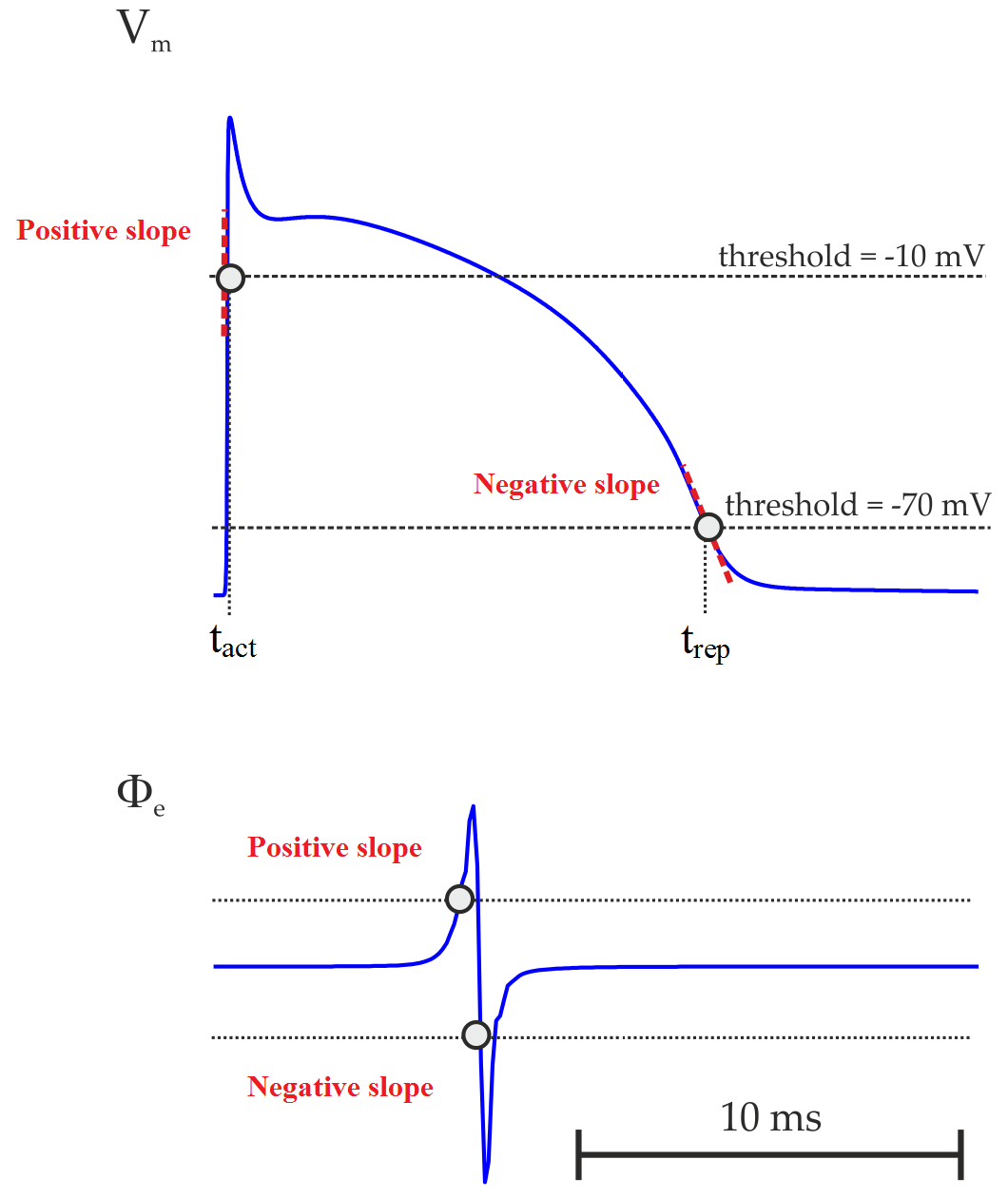Activation (LAT) and repolarization times $$t_{\mathrm{act}}$$ and $$t_{\mathrm{rep}}$$ computed using the threshold crossing method. $$APD = t_{\mathrm{rep}} - t_{\mathrm{act}}$$. ## Problem Setup This example will run a simulation using a 2D sheet model (1 cm x 1 cm). A transmembrane stimulus current of 100 uA/cm^2 is applied for a period of 1 ms at the middle of the left side of the tissue. A ground electrode is placed at the opposite side of the stimulating electrode. Simulation of electrical activity is based on the bidomain equations. Dynamics of the cardiac myocytes within the tissue are described using the Modified Beeler-Router Drouhard-Roberge (DrouhardRoberge) model. Activation times are obtained either from $$V_{\mathrm m}$$ or $$\phi_{\mathrm e}$$ using a threshold crossing method, whereas repolarization can only be obtained by threshold crossing from $$V_{\mathrm m}$$. Estimation of repolarization times from $$\phi_{\mathrm e}$$ is out of the scope of this example. ## Usage To run this example, execute: cd${TUTORIALS}/02_EP_tissue/08_lats

./run.py --help 

The following optional arguments are available (default values are indicated):

--quantity          Quantity/variable/signal used to compute activation time
Options: {vm,phie}
Default: vm
--act-threshold     The threshold value for determining activation from vm or phie
Default: -10 mV
--rep-threshold     The threshold value for determining repolarization from vm
Default: -70 mV
--show-APDs         Visualize APDs instead of LATs

If the program is run with the --visualize option, meshalyzer will automatically load the computed activation sequence (LATs). If the --show_APDs is given, then the computed APDs will be loaded:

Visualize LATs:
./run.py --visualize

Visualize APDs:
./run.py --show-APDs

## Interpreting Results

Differences between activation sequences computed from $$V_{\mathrm m}$$ or $$\phi_{\mathrm e}$$ and/or with different thresholds can be appreciated with meshalyzer (--visualize). The activation sequence for the default parameters looks like this: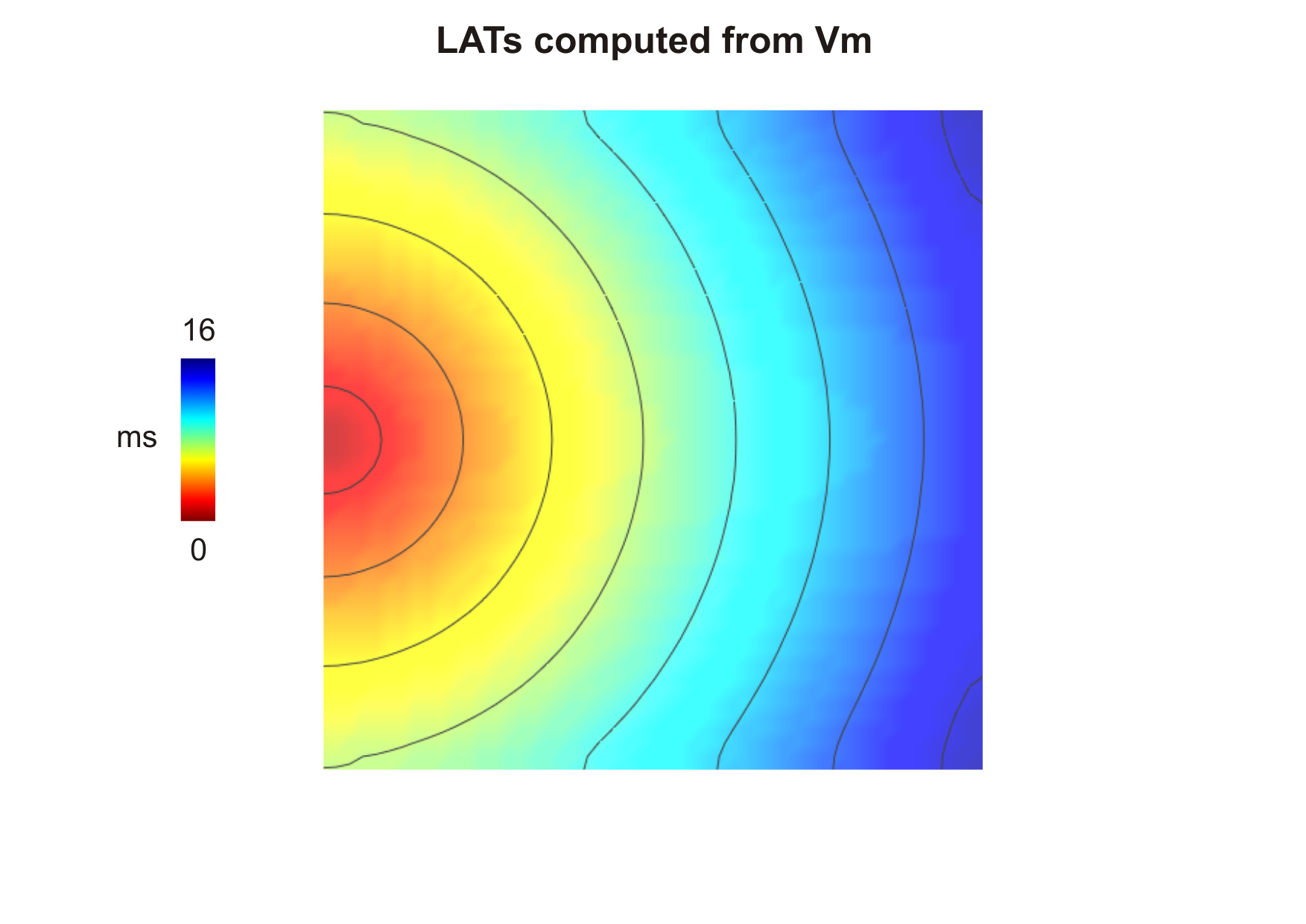Activation sequence computed from $$V_{\mathrm m}$$ (--act-threshold=-10) obtained after delivering a stimulus current in the middle of the left side tissue.

Note

• It is important to note that while computing activation from $$V_{\mathrm m}$$ -lats[].mode has to be set to zero. This is because $$V_{\mathrm m}$$ is about -85 mV in cardiac cells at rest and rapidly rises to about +40 mV during the activation phase. This means $$V_{\mathrm m}$$ will cross the threshold with a positive slope. While computing repolarization, on the other hand, $$V_{\mathrm m}$$ goes from positive values back to the rest. The threshold will be then crossed with a negative slope.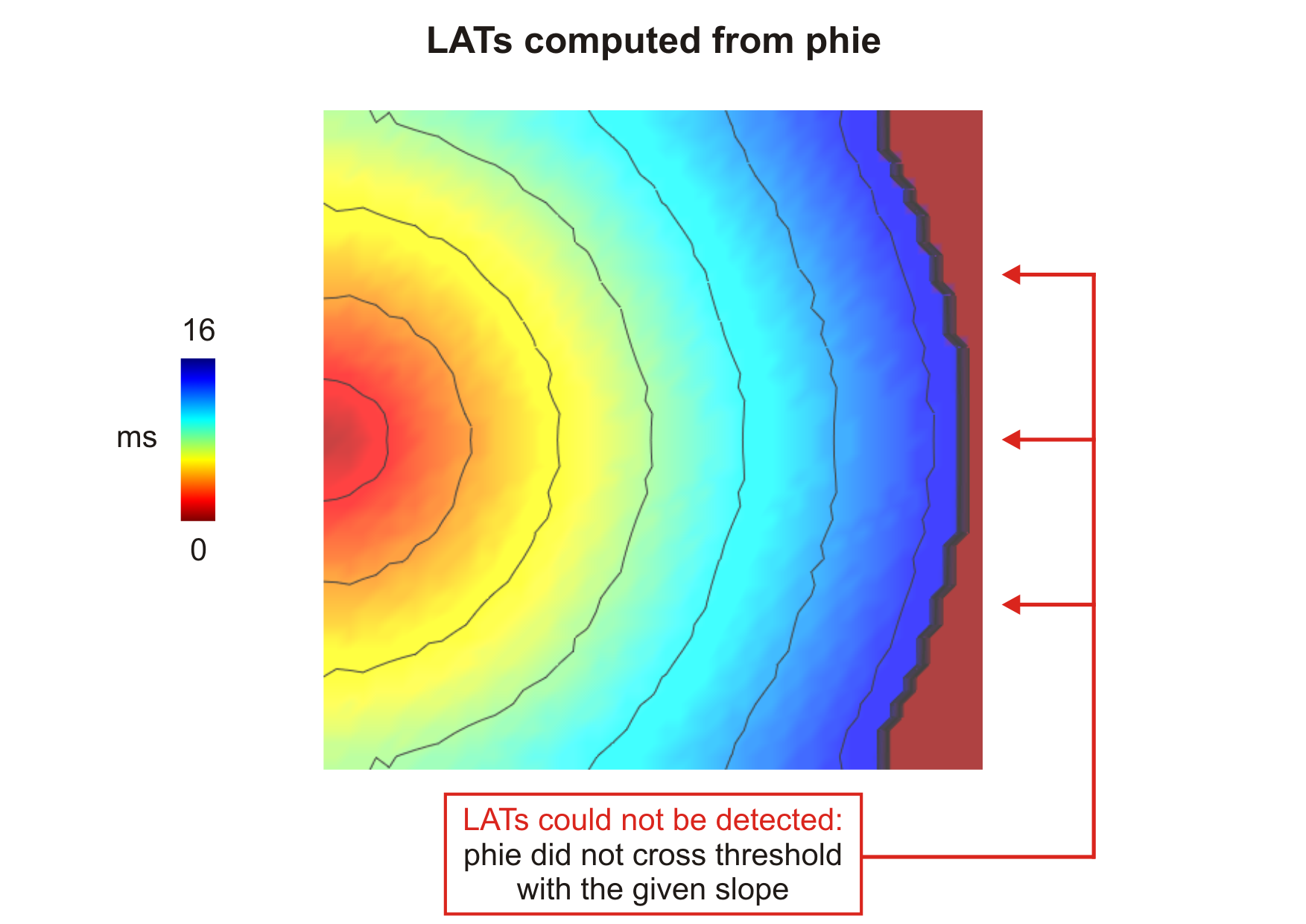Activation sequence computed from $$\phi_{\mathrm e}$$ (--act-threshold=-10) obtained after delivering a stimulus current in the middle of the left side tissue.

Note

• Unlike the shape of $$V_{\mathrm m}$$ which remains pretty much the same in all cells, $$\phi_{\mathrm e}$$ morphology may vary depending on its position in relation to both stimulation and ground electrodes. Thus, LATs obtained based on $$\phi_{\mathrm e}$$ might differ from those obtained from $$V_{\mathrm m}$$.
• If the criteria for threshold crossing detection is never satisfied, the resulting entry in the output file will be -1. See action sequence obtained from $$\phi_{\mathrm e}$$.

The computed APDs using the default parameters looks like this (use --show-APDs instead of --visualize):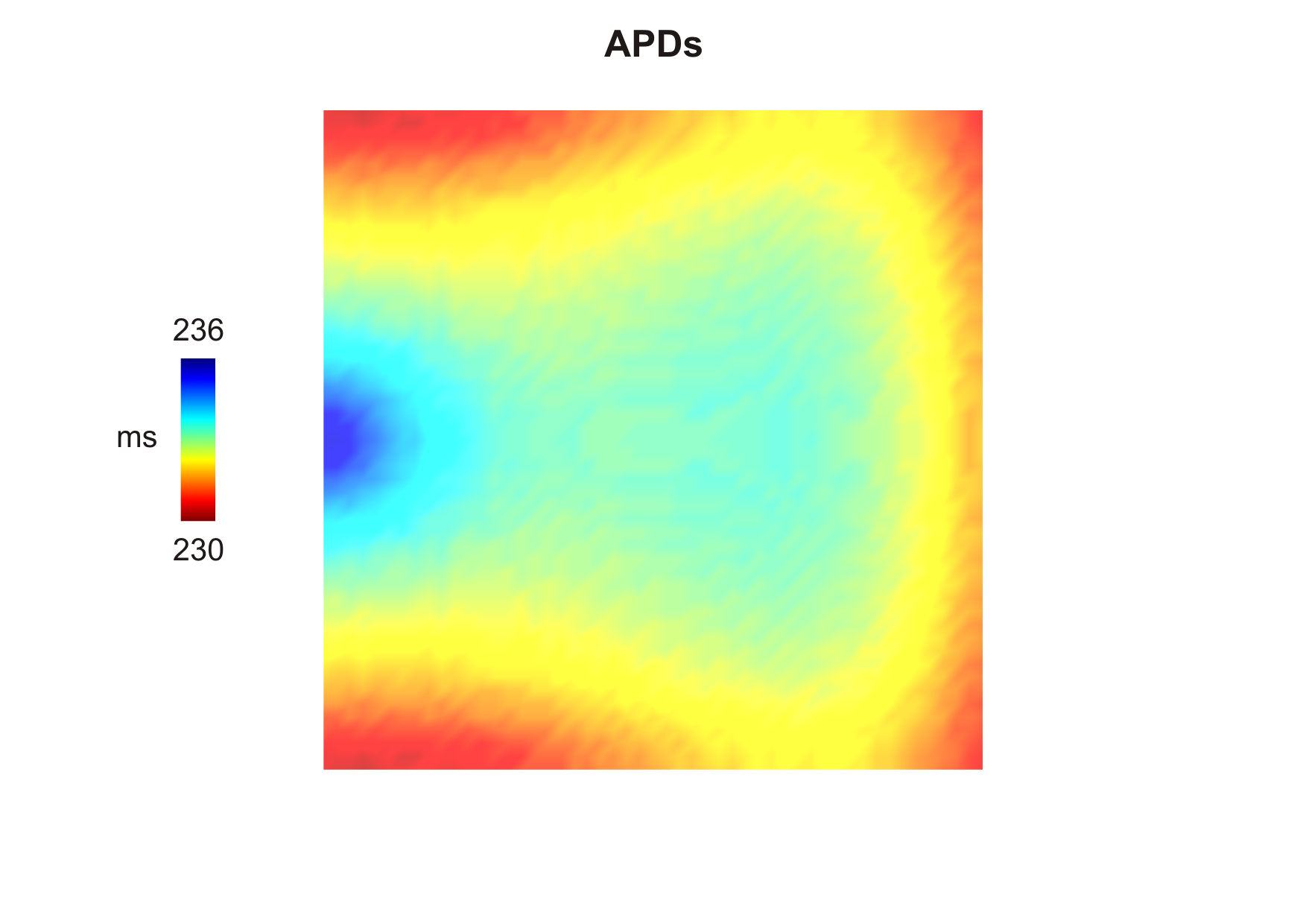Action potential durations computed using the default thresholds (--act-threshold=-10 and --rep-threshold=-70) after delivering a stimulus current in the middle of the left side of the tissue.

## openCARP Parameters

The relevant parameters used in the tissue-scale simulation are shown below:

# Number of events to detect
-num_LATs  = 2

# Event 1: activation
-lats.ID         = 'ACTs'
-lats.all        = 0
-lats.measurand  = 0
-lats.threshold  = -10
-lats.mode       = 0

# Event 2: repolarization
-lats.ID         = 'REPs'
-lats.all        = 0
-lats.measurand  = 0
-lats.threshold  = -70
-lats.mode       = 1

num_LATs referes to the number of events we want to detect. In this example there are two events: activation and repolarization. -lats are options to compute activation while -lats are options to compute repolarization. -lats[].ID is the name of output file; -lats[].all tells openCARP if it should detect only the first event (-lats[].all = 0), i.e., first beat, of all of them (-lats[].all = 1); -lats[].measurand refers to the quantity: $$V_{\mathrm m}$$ (0) or $$\phi_{\mathrm e}$$ (1); -lats[].threshold is the threshold value for determining the event; finally, -lats[].mode tells openCARP to look for threshold crossing with a either a positive or negative slope.

© Copyright 2020 openCARP project    Supported by DFG and EuroHPC    Contact    Imprint and data protection